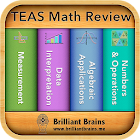TEAS Math Review

All Android applications categories

All Android games categories# TEAS Math Review

0 0

## Screenshots

Description

TEAS Math Review app contains quick revision notes and tons of questions to help you preparing for the TEAS test and get into the nursing school of your choice.

This app covers 51 topics. Each topic has quick revision notes and multiple questions. Questions are similar to the ones asked in TEAS common entrance exams set by ATI. They vary in difficulty levels ranging from 1 to 10.

These 51 types are categorized as follows.

• Numbers & Operations

1. Order of Operations and PEDMAS
2. Multiplication and Division Problems
3. Decimals - Multiplication and Division 1
4. Decimals - Multiplication and Division 2
5. Approximation of Roots and Exponents
6. Improper Fractions and Mixed Numbers
8. Subtracting Fractions
9. Multiplying Fractions
10. Dividing Fractions
11. Fraction Problems in Words
12. Rational Numbers
13. Percent 1
14. Percent 2
15. Equivalent Percents Fractions and Decimals
16. Percent Problems in Words
17. Ratios and Fractions
18. Ratio Problems in Words
19. Direct Proportion
21. Compound Interest

• Algebraic Applications

22. Addition and Subtraction of Algebraic Expressions
23. Multiplication of Algebraic Expressions
24. Algebraic Fractions
25. Translation into Algebraic Expressions
26. Evaluating Expressions 1
27. Evaluating Expressions 2
28. Linear Equations 1
29. Linear Equations 2
30. Linear Equations 3
31. Setting up Equations
32. Absolute Value
33. Inequalities
34. Solving Inequalities
35. Systems of Equations (Linear)
36. Setting up Systems of Equations

• Data Interpretation

37. Frequency Tables
38. Grouped Data
39. Two Way Tables
40. Box Plots
41. Histograms
42. Bar Graphs
43. Pie Charts
44. Line Graphs
45. Scatter Graphs
46. Basic Probability
47. Independent Events

• Measurement

48. Units and Measurements
49. Estimating measurements
50. Converting Units
51. Time and Timetables

DETAILED TEST RESULTS

On the completion of each test the user is given a summary of questions answered correctly, incorrectly and skipped along with the time taken on each question. Every question can be reviewed and all questions have an explanation of the answer.

PROGRESS METER

An interactive pie chart displays current progress for each topic. You can touch the different color slices of the pie and choose if you wish to answer only incorrectly answered questions, unattempted or correctly answered questions. This feature is very useful to achieve 100% score in each topic.

MOCK TEST
After you have practiced all types of questions, you can take a Mock Test with 50 questions. The Mock Test has questions selected randomly from each topic. Scores for the mock tests taken in the past are displayed in a bar chart. This is a great way to track how you are progressing in mock tests. There is no limit on how many mock tests you can take.

FEATURE LIST

• Covers all 51 commonly found types of questions.
• 509 multiple-choice questions.
• Explanation with every question.
• Option to choose the number of questions to test.
• Progress meter helps to track progress, topic wise and mock test wise.
• Ability to re-attempt only the questions you get wrong or correct or never attempted
• Option to choose reset the progress and start from scratch.

Tags: teas algebraic applications